Courses

# Test: Oscillations 2 - From Past 28 Years Questions

## 30 Questions MCQ Test Physics 28 Years Past year papers for NEET/AIPMT Class 11 | Test: Oscillations 2 - From Past 28 Years Questions

Description
This mock test of Test: Oscillations 2 - From Past 28 Years Questions for NEET helps you for every NEET entrance exam. This contains 30 Multiple Choice Questions for NEET Test: Oscillations 2 - From Past 28 Years Questions (mcq) to study with solutions a complete question bank. The solved questions answers in this Test: Oscillations 2 - From Past 28 Years Questions quiz give you a good mix of easy questions and tough questions. NEET students definitely take this Test: Oscillations 2 - From Past 28 Years Questions exercise for a better result in the exam. You can find other Test: Oscillations 2 - From Past 28 Years Questions extra questions, long questions & short questions for NEET on EduRev as well by searching above.
QUESTION: 1

### A particle moving along the X-axis, executes simple harmonic motion then the force acting on it is given by 

Solution:

For simple harmonic motion, F = – kx.
Here,  k = Ak.

QUESTION: 2

### A particle, with restoring force proportional to displacement and resistve force proportional to velocity is subjected to a force F sin ω0. If the amplitude of the particle is maximum for ω = ω1 and the energy of the particle is maximum for ω = ω2, then [1989,1998]

Solution:

At maximum energy of the particle, velocity resonance takes place, which occurs when frequency of external periodic force is equal to natural frequency of undamped vibrations, i.e. ω2 = ω0.
Further, amplitude resonance takes place at a frequency of external force which is less than the frequency of undamped natural vibrations, i.e. ω1 ≠  ω0 .

QUESTION: 3

### A mass m is suspen ded from a two coupled springs, connected in series. The force constant for springs are k1 and k2. The time period of the suspended mass will be 

Solution:

The effective spring constant of two springs in series;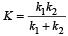Time period,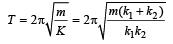QUESTION: 4

The composition of two simple h ar monic motions of equal periods at right angle to each other and with a phase difference of π results in the displacement of the particle along 

Solution:

x = a sinωt and y = b sin (ωt + π) = – b sinωt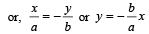It is an equatation of a straight line.

QUESTION: 5

The angular velocity and the amplitude of a simple pendulum is ω and a respectively. At a displacement x from the mean position if its kinetic energy is T and potential energy is V, then the ratio of T to V is 

Solution:

PE ,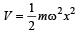and K.E.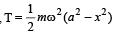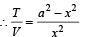QUESTION: 6

A body is executing S .H. M . When the displacements from the mean position are 4cm and 5 cm, the corresponding velocities of the body are 10 cm per sec and 8 cm per sec. Then the time period of the body is 

Solution:

For S.H.M., Velocity,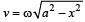at displacement x.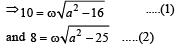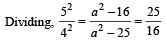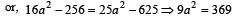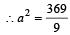Putting this value in equation (2) mentioned above,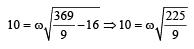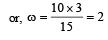radian / sec .

Time period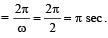QUESTION: 7

A simple pendulum is suspended from the roof of a trolley which moves in a horizontal direction with an acceleration a, then the time period is given by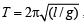, where g is equal to 

Solution:

The effective value of acceleration due to gravity is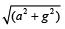QUESTION: 8

If a simple harmonic oscillator has got a displacement of 0.02 m and acceleration equal to 2.0 m/s2 at any time, the angular frequency of the oscillator is equal to 

Solution: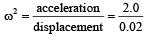ω2= 100 or ω = 10 rad/s

QUESTION: 9

A simple harmonic oscillator has an amplitude A and time period T. The time required by it to travel from x = A to x = A/2 is 

Solution:

For S.H.M.,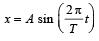When x = A,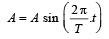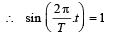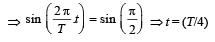When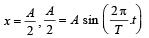or,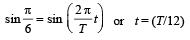Now, time taken to travel from x = A to x = A/2 is  (T/4 – T/12) = T/6

QUESTION: 10

A wave has S.H.M (Simple Harmonic Motion) whose period is 4 seconds while another wave which also possess SHM has its period 3 seconds. If both are combined, then the resultant wave will have the period equal to 

Solution:

Beats are produced. Frequency of beats will be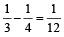Hence time period = 12 s.

[Alt : Frequency of combined S.H.M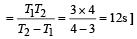QUESTION: 11

A body executes S.H.M with an amplitude A. At what displacement from the mean position is the potential energy of the body is one fourth of its total energy ? 

Solution: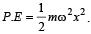Total energy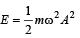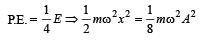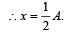[Alt : The displacement at which the P.E. becomes n times the total energy is given by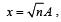where A is the amplitude.]

QUESTION: 12

Which one of the following is a simple harmonic motion? 

Solution:

A wave moving through a string fixed at both ends, is a transverse wave formed as a result of simple harmonic motion of particles of the string.

QUESTION: 13

A par ticle is subjected to two mutually perpendicular simple harmonic motions such that its x and y coordinates are given by

x = 2 sin ωt;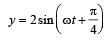The path of the particle will be 

Solution:

As phase difference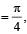the resultant path of particle is an ellipse.

QUESTION: 14

In a simple harmonicmotion, when the displacement is one-half the amplitude, what fraction of the total energy is kinetic? 

Solution:

Total energy of particle executing S.H.M. of amplitude (A).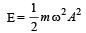K.E.of the particle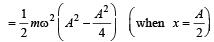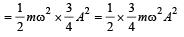Clearly ,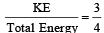[Alt : If the displacement be n times the amplitude, then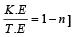QUESTION: 15

A linear harmonic oscillator of force constant 2 × 106 N/m and amplitude 0.01 m has a total mechanical energy of 160 J. Its 

Solution:

k = 2 × 106 N/m
Amplitude (A) = 0.01 m
Total mechanical energy = 160J
As we know that total mechanical energy is equal to total maximum potential energy.
Maximum K. E.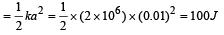QUESTION: 16

A particle starts simple harmonic motion from the mean position. Its amplitude is A and time period is T.What is its displacement when its speed is half of its maximum speed 

Solution: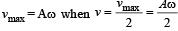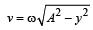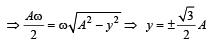[Alt : The displacement at which the speed is n times the maximum speed is given by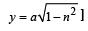QUESTION: 17

If the length of a simple pendulum is increased by 2%, then the time period 

Solution:

We know that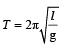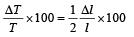If length is increased by 2%., time period increases by 1%.

QUESTION: 18

Two  simple harmonic motions with the same frequency act on a particle at right angles i.e., along x and y axis. If the two amplitudes are equal and the phase difference is ω/2, the resultant motion will be 

Solution:

Equation of two simple harmonic motions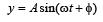....(1)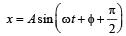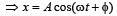....(2)

On squaring and adding equations  (1) and (2),
x2 + y2 = A2

This is an equation of a circle. Hence, resulting motion will be a circular motion.

QUESTION: 19

A hollow sphere is filled with water. It is hung by a long thread. As the water flows out of a hole at the bottom, the period of oscillation will 

Solution:

Time period of simple pendulum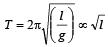where l is effective
length. [i.e distance between centre of suspension and centre of gravity of bob]

Initially, centre of gravity is at the centre of sphere. When water leaks the centre of gravity goes down until it is half filled; then it begins to go up and finally it again goes at the centre. That is effective length first increases and then decreases. As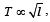, so time period first increases and then decreases.

QUESTION: 20

Two simple pendulums of length 5m and 20m respectively are given small linear displacement in one direction at the same time. They will again be in the phase when the pendulum of shorter length has completed ....... oscillations 

Solution:

Let the pendulums be in phase after t sec of start. Within this time, if the bigger pendulum executes n oscillations, the smaller one will have executed (n + 1) oscillations.
Now, the time of n oscillation =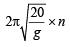& the time of (n + 1) oscillation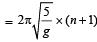To be in phase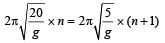or,      2n = n + 1
or,      n = 1
Hence, the no. of oscillations executed by shorter pendulum = n + 1 = 1 + 1 = 2

QUESTION: 21

A mass m is vertically suspended from a spring of negligible mass; the system oscillates with a frequency n. What will be the frequency of the system, if a mass 4 m is suspended from the same spring? 

Solution: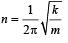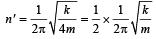On putting the value of n  we get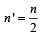QUESTION: 22

The time period of a simple pendulum is 2 seconds. If its length is increased by 4-times, then its period becomes 

Solution: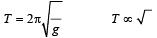If  is increased by 4 times, time period will increase by two times.

QUESTION: 23

A particle executing S.H.M. has amplitude 0.01m and frequency 60 Hz. The maximum acceleration of the particle is 

Solution:

Amplitude (A) = 0.01 m, Frequency = 60 Hz
Maximum acceleration = Aω2 = 0.01 x (2πn)2
= 0.01 x 4π2 x 60 x 60 = 144π2 m/sec2

QUESTION: 24

Masses MA and MB hanging from the ends of strings of lengths LA and LB are executing simple harmonic motions. If their frequencies are fA = 2fB, then 

Solution: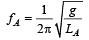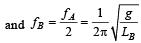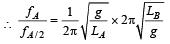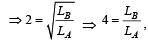regardless of mass.

QUESTION: 25

Two simple harmonic motions act on a particle.
These harmonic motions are x = A cos (ωt + δ), y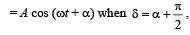the resulting motion is 

Solution: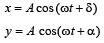....(1)

When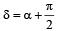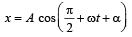x = -A sin (ωt + α)            ....(2)
Squaring (1) and (2)
and then adding x2 + y2 = A2 [cos2 (ωt +α) + sin2 (ωt +α)] or x2 + y2 = A2, which is the equation of a circle.
The present motion is anticlockwise.

QUESTION: 26

A simple pendulum has a metal bob, which is negatively charged. If it is allowed to oscillate above a positively charged metallic plate, then its time period will 

Solution:

We know that the time period (T)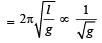Since the negatively charged bob is attracted by the positively charged plate, therefore acceleration due to gravity will increase and time period will decrease.

QUESTION: 27

There is a body having mass m and performing S.H.M. with amplitude a. There is a restoring force F = –kx. The total energy of body depends upon

Solution:

Total Energy of body perfor ming simple harmonic motion =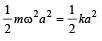where k = mω2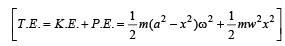Hence energy depends upon amplitude and k (spring constant).

QUESTION: 28

A body of mass M, executes vertical SHM with periods t1 and t2, when separately attached to spring A and spring B respectively. The period of SHM, when the body executes SHM, as shown in the figure is t0. Then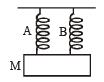Solution:⇒  k = Const.t-2

Here the springs are joined in parallel. So k0 = k1+k2 where k0 is resultant force constant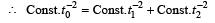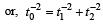QUESTION: 29

The amplitude of a pendulum executing damped simple harmonic motion falls to 1/3 the original value after 100 oscillations. The amplitude falls to S times the original value after 200 oscillations, where S is 

Solution:

In damped harmonic oscillator, amplitude falls exponentially.

After 100 oscillations amplitude falls to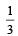times.

∴ After next 100 oscillations i.e., after 200 oscillations amplitude falls to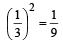times.

QUESTION: 30

A particle is executing a simple harmonic motion of amplitude a. Its potential energy is maximum when the displacement from the position of the maximum kinetic energy is 

Solution:

P.E. of particle executing S.H.M. =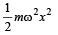At x = a, P.E. is maximum i.e.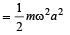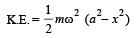At x = 0, K.E. is maximum.

Hence, displacement from position of maximum Kinetic energy = ±a.